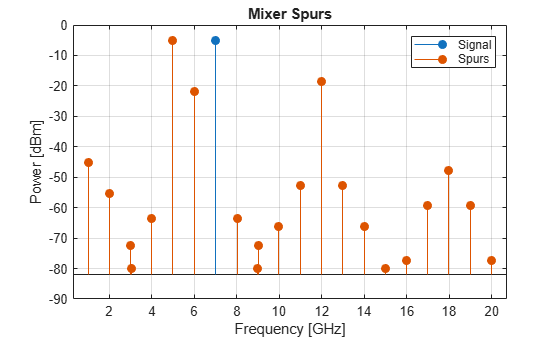# mixerIMT

## Description

Use the `mixerIMT` object to perform frequency translation defined in an intermodulation table (IMT) for a single-tone carrier mixed with a local oscillator (LO) signal. If you design an RF system using an `rfbudget` object or the RF Budget Analyzer app you can model an IMT mixer with the `mixerIMT` object and add it to the RF system. You can then export this element to RF Blockset™ or to an `rfsystem` System object™ for circuit envelope analysis.

## Creation

### Syntax

``imt = mixerIMT``
``imt = mixerIMT(Name=Value)``

### Description

````imt = mixerIMT` creates an IMT mixer object with default property values.```

example

````imt = mixerIMT(Name=Value)` sets Properties using one or more name-value arguments. For example, ```imt = mixerIMT(LO=5e6)``` sets the local oscillator frequency of an IMT mixer to 5 GHz. Properties not specified retain their default values.```

## Properties

expand all

Name of the IMT mixer, specified as a string scalar or character vector.

Example: `Name='imtmixer'`

Data Types: `char` | `string`

Reference input power, specified as a scalar in dBm.

Example: `'ReferenceInputPower=-10`

Data Types: `double`

Nominal output power, specified as a scalar in dBm.

Example: `NominalOutputPower=8`

Data Types: `double`

Noise figure, specified as a scalar in dBm.

Example: `NF=8`

Data Types: `double`

Local oscillator frequency, specified as a real finite positive scalar in Hz.

Example: `LO=2e9`

Data Types: `double`

Conversion direction, specified as either `'Up'` or `'Down'`.

Example: `ConverterType='Down'`

Data Types: `string` | `char`

Input impedance, specified as a positive scalar in ohms.

Example: `Zin=40`

Data Types: `double`

Output impedance, specified as a positive scalar in ohms.

Example: `Zout=40`

Data Types: `double`

IMT spurs to plot, specified as a real square matrix.

Example: `IMT=[99 99 80; 99 0 99; 99 80 99]`

Use S2D file to design IMT mixer, specified as a numeric or logical ```1 (true)``` or `0 (false)`.

Example: `UseDataFile=1`

Data Types: `logical`

File name of the S2D file, specified as a string scalar or a character vector.

Example: `FileName='samplespur1.s2d'`

#### Dependency

To enable this property, set `UseDataFile` to `1`.

Data Types: `string` | `char`

Number of input and output ports, returned as a positive scalar.

Data Types: `double`

Terminals of the IMT mixer, returned as a cell array of strings.

Data Types: `string`

## Object Functions

 `sparameters` Calculate S-parameters for RF data, network, circuit, and matching network objects `rfplot` Plot mixer spurs of IMT mixer

## Examples

collapse all

Create an IMT mixer with the LO frequency of 5995 MHz and with the IMT spurs.

```imt = mixerIMT(LO=5995e6,IMT=... [99 17 13.6 42.9; 40 0 47.7 54.3; 50.4 58.5 61.3 72.2; 75 67.4 75 99]);```

Plot the mixer spur at an input signal frequency of 1005 MHz.

`rfplot(imt,1005e6)`## Version History

Introduced in R2022a

expand all